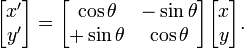# javascript图像处理―仿射变换深度理解

更新时间：2013年01月16日 09:06:37   作者：cos（α+β）=cosαcosβ-sinαsinβ

sin（α+β）=sinαcosβ+cosαsinβ或者var getRotationArray2D = function(__angle, __x, __y){
var sin = Math.sin(__angle) || 0,
cos = Math.cos(__angle) || 1,
x = __x || 0,
y = __y || 0;

return [cos, -sin, -x,
sin, cos, -y
];
};

var warpAffine = function(__src, __rotArray, __dst){
(__src && __rotArray) || error(arguments.callee, IS_UNDEFINED_OR_NULL/* {line} */);
if(__src.type && __src.type === "CV_RGBA"){
var height = __src.row,
width = __src.col,
dst = __dst || new Mat(height, width, CV_RGBA),
sData = new Uint32Array(__src.buffer),
dData = new Uint32Array(dst.buffer);

var i, j, xs, ys, x, y, nowPix;

for(j = 0, nowPix = 0; j < height; j++){
xs = __rotArray * j + __rotArray;
ys = __rotArray * j + __rotArray;
for(i = 0; i < width; i++, nowPix++, xs += __rotArray, ys += __rotArray){

if(xs > 0 && ys > 0 && xs < width && ys < height){

y = ys | 0;
x = xs | 0;

dData[nowPix] = sData[y * width + x];
}else{
dData[nowPix] = 4278190080; //Black
}
}
}
}else{
error(arguments.callee, UNSPPORT_DATA_TYPE/* {line} */);
}
return dst;
};